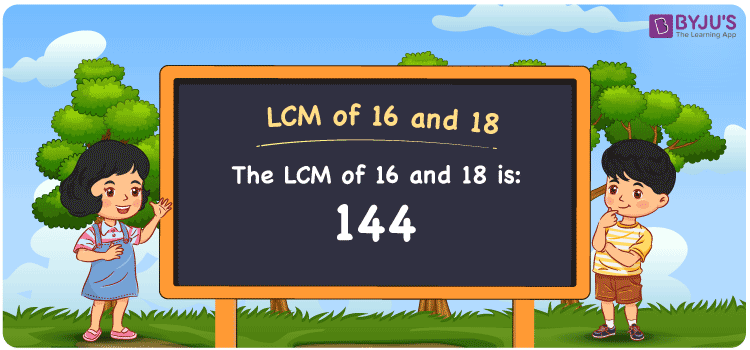Checkout JEE MAINS 2022 Question Paper Analysis : Checkout JEE MAINS 2022 Question Paper Analysis :

# LCM of 16 and 18

LCM of 16 and 18 is 144. The smallest common multiple which is divisible evenly by the two given numbers is the LCM. Students can find the least common multiples of 16 and 18 from the common multiples. (16, 32, 48, 64, 80, 96, 112, ….) and (18, 36, 54, 72, ….) are the multiples of 16 and 18. The LCM of two numbers is determined with the help of methods like listing the multiples, prime factorization and division method.

## What is LCM of 16 and 18?

The answer to this question is 144. The LCM of 16 and 18 using various methods is shown in this article for your reference. The LCM of two non-zero integers, 16 and 18, is the smallest positive integer 144 which is divisible by both 16 and 18 with no remainder.## How to Find LCM of 16 and 18?

LCM of 16 and 18 can be found using three methods:

• Prime Factorisation
• Division method
• Listing the multiples

### LCM of 16 and 18 Using Prime Factorisation Method

The prime factorisation of 16 and 18, respectively, is given by:

16 = 2 x 2 x 2 x 2 = 2

18 = 2 x 3 x 3 = 2¹ x 3²

LCM (16, 18) = 144

### LCM of 16 and 18 Using Division Method

We’ll divide the numbers (16, 18) by their prime factors to get the LCM of 16 and 18 using the division method (preferably common). The LCM of 16 and 18 is calculated by multiplying these divisors.

 2 16 18 2 8 9 2 4 9 2 2 9 3 1 9 3 1 3 x 1 1

No further division can be done.

Hence, LCM (16, 18) = 144

### LCM of 16 and 18 Using Listing the Multiples

To calculate the LCM of 16 and 18 by listing out the common multiples, list the multiples as shown below

 Multiples of 16 Multiples of 18 16 18 32 36 48 54 64 72 80 90 96 108 112 126 128 144 144 162

LCM (16, 18) = 144

## Video Lesson on Applications of LCM## LCM of 16 and 18 Solved Examples

What is the smallest number exactly divisible by 16 and 18?

Solution:

We know that

LCM is the smallest number exactly divisible by 16 and 18.

Multiples of 16 = 16, 32, 48, 64, 80, 96, 112, 128, 144, ….

Multiples of 18 = 18, 36, 54, 72, 90, 108, 126, 144, ….

Hence, the LCM of 16 and 18 is 144.

## Frequently Asked Questions on LCM of 16 and 18

### How to find the LCM of 16 and 18?

The LCM of 16 and 18 can be found using the following methods

Prime Factorisation

Division method

Listing the multiples

### Use the prime factorisation method and find the LCM of 16 and 18.

To get the LCM, we should know the factors

16 = 2 x 2 x 2 x 2 = 2⁴

18 = 2 x 3 x 3 = 2¹ x 3²

LCM is the product of prime factors raised to the highest exponent among 16 and 18.

LCM of 16 and 18 = 144

### Find the GCF if the LCM of 16 and 18 is 144.

LCM x GCF = 16 x 18

As the LCM = 144

144 x GCF = 288

GCF = 288/144 = 2